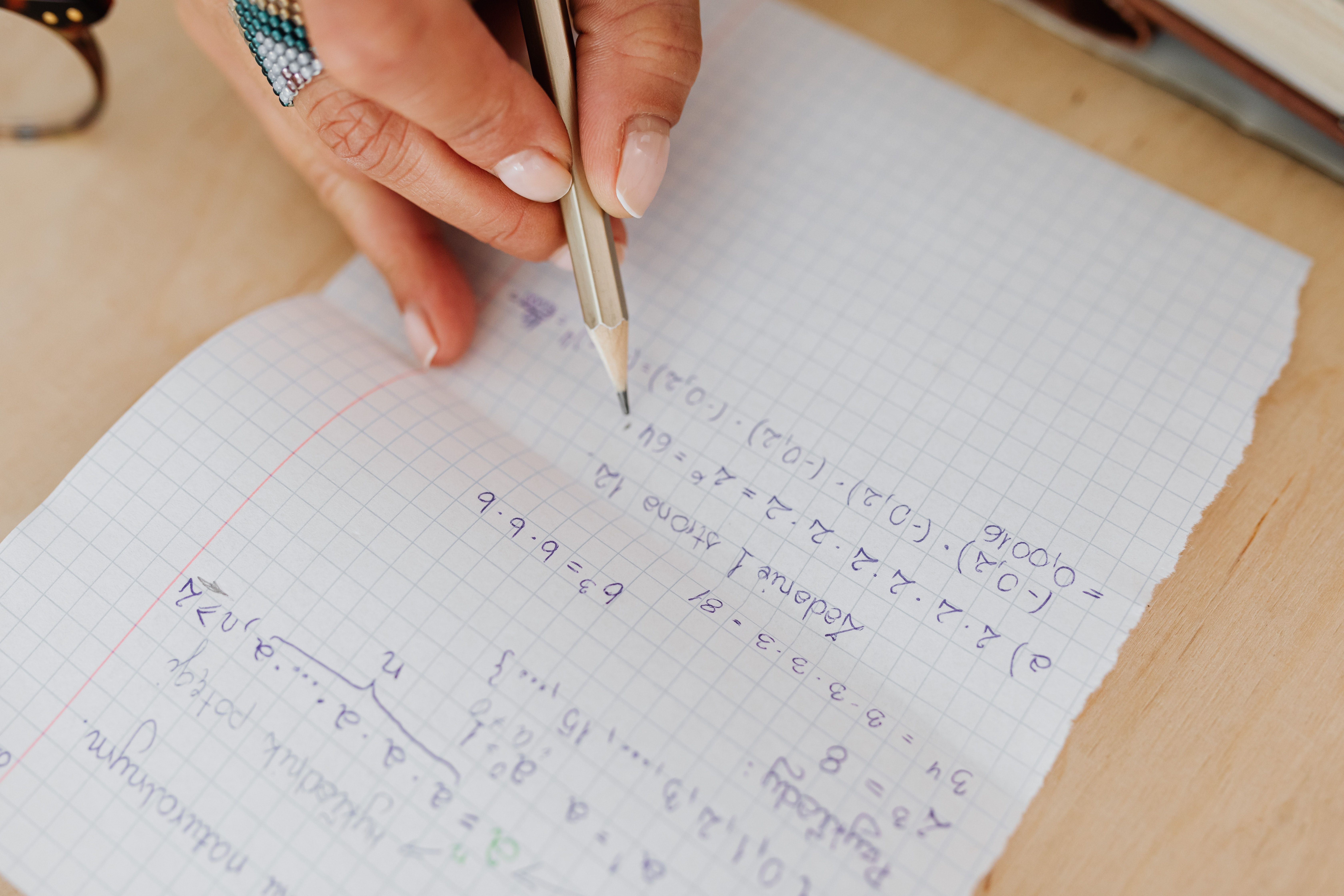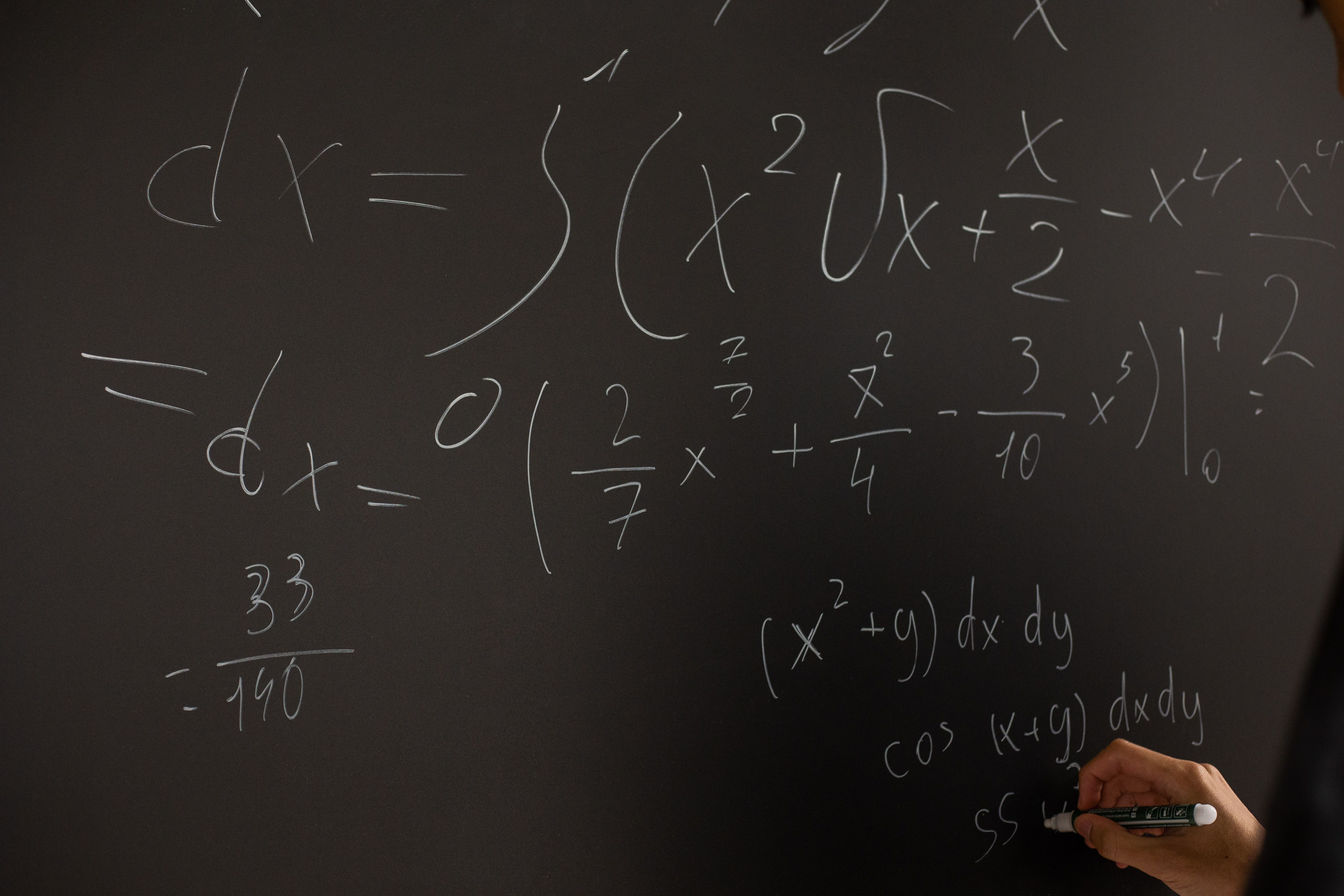Find a tutor# Common Algebra Mistakes And How to Avoid Them

Part one of the essential guide to eliminating errors that trip up many test-takers.Jenna K.
Math
Study Tips

Whether you are a beginning algebra student, or a confident calculus whiz, students of all levels make these and similar errors. To avoid these kinds of errors and have a better understanding of fundamental math concepts, think carefully about the reason someone might make such a mistake, as well as the reason the correct approach is, in fact, correct. You’ll grow as a math student and overcome a common trap: memorizing a series of steps to take to solve an individual problem.

## 1. Sloppy use of mathematical symbols

Mathematics has its own language, and symbols have very specific meanings. If we use the wrong “words,” we can end up expressing mathematical statements that are either ambiguous or incorrect. This can be confusing not only to you, but also to a teacher evaluating your answers on homework or a test. Here are some examples of common errors students make when using mathematical symbols:### Misusing equals signs

The equals sign in math has two very specific functions. It can either be used to state that two mathematical expressions are equal in value (as in, $2x = 4$), or it can be used to make a definition (as in $f(x) = x^2$. Be careful about using the equals sign in other ways, such as when you are working through a series of computations to signal successive steps in the computation. For example, I have seen students write things like this:

$2x=4=x=2$

or this:

$2x = 4$

$= x=2$

A student who writes the above equation is trying to use the equal sign to mean “therefore.” Instead, try using an arrow: $2x=4 → x=2$. This makes it clear to a reader what the logical relationship is between each of the expressions.

### Implicit and/or dropped parentheses

Writing in parentheses in a mathematical statement can reduce your chance of errors, when there is an implicit order of operations, or an implicit set of parentheses.

Consider a fraction, say, $\frac{2x+3}{5}$​. We know that, if we were to evaluate the fraction, we would have to evaluate the numerator before dividing by 5. Instead of leaving this order of operations implicit, make it clear by writing in the parentheses: $\frac {2x+3} {5} =\frac {(2x+3)} {5}$​. Otherwise, you may make a mistake in how you solve problems using that algebraic expression. E.g., $\frac{6x}{5}-\frac{2x+3}{5}= \frac{4x-3}{5}$ NOT $\frac{4x+3}{5}$​.

Other examples of implicit/dropped parentheses:

• Suppose $f(x)= x-3. Then, 5-f(x)= 5 - (x - 3) = 8 - x$NOT $5 - x - 3$. Even though we didn’t use parentheses around $f(x)$, in the first step we need to write them around $(x - 3)$ when we substitute to indicate that this binomial all together represents a single thing (namely, the function $f$).
• Suppose $g(x)= 2-x^2$. Then, $g(4y)= 2 - 16y^2$, NOT $2 - 4y^2$.

### Writing fractions and square roots unambiguously

I always encourage students to write fractions in the $\frac{a}{b}$​ format, instead of the $a/b$ format. Consider $2/3x$. It’s not clear whether this means $2/(3x)$ or $(2/3)x$, which is a significant difference.

When you’re writing roots, make sure the top line completely extends over everything that belongs within the radical. The expression $\sqrt{3}x$ means something very different from $\sqrt{3x}$​, so make sure you write them to look different! In fact, it's best to write anything that is multiplied by a root but doesn’t belong inside the radical in front of the root: $x\sqrt{3}$ is clearer than $\sqrt{3}x$.

## 2. Cancelling error

You may be used to cancelling common factors on both sides of an equals sign when you are solving an equation (if $2x = 4$, divide both sides by 2 to get the answer). However, this can lead to errors when the common factor is not a number but an expression involving a variable. There may be multiple solutions which you would miss if you simplify the equation in this way.

Consider $x^3 = x$. You might be tempted to cancel $x$ on both sides to get $x^2 = 1$. The problem with this is, since you don’t yet know what $x$ is, you might have just divided by zero! Looking at the equation, it’s clear that $x = 0$ is a possible solution, so we have to consider this case when we solve the equation.

Here’s what I would do:

$x^3 = x$ implies EITHER $x = 0$ OR, if $x$ is not zero, then we can divide both sides by $x$ to find $x^2 = 1$, which has solutions $x = ±1$. This gives us a complete solution set: $x = \{0, ±1\}.$

Here’s another example: $x^2 - 9 = x + 3$. If we factor the left-hand side we get $(x + 3)(x - 3) = x + 3$. Then, we acknowledge that $x + 3 = 0$ is a possible solution (i.e., $x = -3$). Otherwise, we can cancel $x + 3$ on both sides to find $x - 3 = 1$ or x = $x = 4$. So, the complete solution set is $x = -3, 4$.## 3. Mistaking roots for solutions

When factoring or using the quadratic formula to solve for the roots of a quadratic polynomial, remember that these options only work if we have an equation of the form $ax^2 + bx + c = 0$, and not if the right hand side is a non-zero value. Solving for the roots of the quadratic $ax^2 + bx + c$ gives us the $x$-values where this quadratic is equal to zero. However, if I had an equation like $ax^2 + bx + c$, I should be looking for the $x$-values where the left-hand side quadratic is equal to 3, not to zero. To fix this, I need to subtract by 3 on both sides to get a quadratic on the left that is equal to zero on the right, and solve for roots from there.

Here’s what’s happening conceptually when I do this: the solutions to $ax^2 + bx + c = 3$ are the points where the quadratic $y = ax^2 + bx + c$intersects the horizontal line $y = 3$. If I move my quadratic downwards by three units, at all the $x$-values where the parabola used to intersect $y = 3$, it will now intersect the $x$-axis,$y$ $y = 0$. By subtracting by three on both sides of my equation, I’m essentially moving my quadratic down by three units while also moving the horizontal line $y = 3$ down to the $x$-axis. The $x$ values of those points of intersection remain the same, since I only shifted things vertically and not horizontally.

Here’s an example: $x^2 - 1 = 3$. The roots of $x^2 - 1$ are $x = ±1$ , where the solutions to this particular equation are $x = ± 2$.

## Final Thoughts

Whew! Great job if you’ve made it this far: you’re on your way to mastering algebraic concepts that trip up many test-takers. Feeling lost? You’re not alone in this. Our brainy algebra tutors have helped countless students nail middle and high school math, so help is close at hand.

In part 2 of this series, we review common mistakes in linear functions, exponents, polynomial fractions and more. Keep practicing!## WORK WITH ONE OF OUR TUTORS

Our team of 50+ brilliant and caring tutors have helped thousands of students gain confidence, improve test scores, and achieve their academic goals!

## Related Blog PostsBy Jenna K.
The Inside Story: Finding the Area of a Circle
Learning why this formula “works” can help you understand math at a deeper level, building your skills for school and tests.By Jenna K.
MORE Common Algebra Mistakes And How to Avoid Them
Part two of the essential guide to eliminating errors that trip up many test-takers.By Jenna K.
Common Algebra Mistakes And How to Avoid Them
Part one of the essential guide to eliminating errors that trip up many test-takers.By Lisa Speransky
Abstract Math Concepts Start in 6th Grade
Let's talk about Middle School Math.